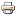# Classroom experiments and activities## Free FallFollowing the steps of Galileo, perform his famous Acceleration Experiment and measure the value of the Earth's gravitational accelerationDescription of experiment.Tomasz Skowron XII High School Szczecin, Poland Janusz Cichor 3rd Gymnasium Augustów, Poland

Enjoyed your successful experiment? Send us euhoupl@euhou.net a description of your setup and .avi file. We will publish the most interesting contributions!

Determining the gravitational acceleration by means of free fall

Tomasz Skowron

XIII LO w Szczecinie

Free fall is a special case of a uniformly accelerated motion, where the acceleration is caused by gravitation, and the initial speed is equal to 0 m/s.

The equation for distance travelled in uniformly accelerated motion takes the following form in the case of free fall:where:
s - the height from which the body is falling;
t - the duration of free falling;
g - the value of gravitational acceleration.

Let us try to find the value of gravitational acceleration by analysing the motion of a body in free fall. We can do this with the help of a steel tape, a tennis ball, a webcam and a computer with SalsaJ - a program that will help us analyse the results of the experiment. To begin with, we record the movement of a falling tennis ball. Secondly, we save the recording as an .avi file, and then we use SalsaJ to convert it into separate frames: Image/Stacks/Convert Stack to Images.

Before you start recording, you need to adjust two elements, i.e. frames per second (I chose 15 frames per second, which gave me one frame every 0.067 s) and in order to have an appropriate number of reference points - the height, from which the ball is falling (in my experiment it was 1.4 m). Knowing the time between subsequent frames, we can find the distance the ball travelled in a given time. The ball is under the influence of gravitation Fg=mg, which causes its uniform acceleration. In equal time intervals the ball will travel ever longer distances, as is shown in the equation we noted down earlier. This fact has been confirmed, the proof being fig.1, which shows the position of the ball in 8 subsequent frames.From these pictures we can read the position of the ball together with the time that passed from the start of the motion. We save these data in the form of a table in a text file.
I presented the results of my experiment in table 1.

 No s (m) t (s) 1 0.1 0.000 2 0.2 0.067 3 0.35 0.134 4 0.55 0.201 5 0.80 0.268 6 1.10 0.335 7 1.40 0.403

I would like to point out, however, that we cannot be sure that the first frame was taken when the position of the ball was s=0, so we cannot assume that t=0 and v0=0 when s=0. This is why I assumed that t=0 when s= 0.1 m, and hence the formula for distance takes the following form:Now we can mark the reference points on a graph and perform curve fitting. To do this we will use SalsaJ again. Select Analyze/Tools/Curve Fitting. Use the dialogue box to open the file with our data and choose Polynomial of degree 2 as the curve fitting method and click Fit. The program will display a graph of the distance / time relation and will give the values of coefficients a, b and c in the quadratic equation, where b represents the initial velocity v0 and c = g/2. In my experiment I obtained the following results: v0=1.32 m/s, gravitational acceleration 9.66 m/s2.

Figure 2 shows the graph of distance / time relation in my experiment. The graph was prepared in Microsoft Excel.The obtained value of gravitational acceleration is different from the known value 9.81 m/s2, but let us remember that measurement error is inevitable in every experiment. As for the time measurement, we can assume that the error was relatively small, but the error in distance measurement was considerable. There are two main reasons for these errors - the diameter of the tennis ball is comparable to the smallest unit on the steel tape, and the fact that when the ball is gaining speed, its trace on the photograph becomes blurred, which makes it difficult to determine its exact position.

However, I still believe we can accept this result as correct, because it reflects correctly the phenomenon we were examining.

I would like to thank professor Aleksander Filip Żarnecki from IFD UW for the idea and his hints on how to perform this experiment.